## 2.3.6 Energy barriers calculations2.4

As exposed in the previous sections the determination of the stability of the magnetic materials needs the evaluation of the energy barrier or equivalently to find the relevant saddle point of the magnetic system. Numerical evaluation of the energy barrier should be done in a multidimensional space and is a difficult problem, especially when collective reversal modes are involved. The calculation of the energy barrier normally involves the search of the minimum energy path, a continuous path that joins two minima and provides the shortest path. An evaluation of energy barrier minimizing the action along the path was suggested in Ref. [Berkov 98]. In chemical physics the problem has been amply studied and several methods have been used, most of them based on elastic band method, an improved version of which is the Nudged Elastic Band method (NEB) that was first applied to the study of the adsorption of hydrogen on Cu surfaces [Mills 95]. In magnetic systems, the use of the nudged elastic band method has been extensively studied in nano-sized systems on the basis of the micromagnetic description [Dittrich 02,Suess 05b]. The method uses a series of images, which move parallel to thedirection, and introduces elastic constants between them. The later is done in order to maintain the separation between the images that otherwise accumulate near the minima resulting in a bad resolution of the saddle point. The saddle point is interpolated from the position of the nearest images, therefore, this kind of methods is known as interpolation methods.

In this thesis the method of the Lagrangian multiplier is used to determine energy barriers. This method can be used in simple magnetic elements such as nano-sized magnetic grains, particles, dots, wires etc. In these systems the occurrence of only one or several reversal modes could be expected. Consequently, the multidimensional space could be parametrized as a function of one reaction coordinate'', as, for example, average magnetization vector. Previously, this method has been proposed and successfully applied to determine the effective energy landscape and energy barriers of small magnetic particles with surface anisotropy [Garanin 03]. The implementation of the method on the basis of existing codes with energy minimization, using, for example, the Landau-Lifshitz-Gilbert equation integration is much simpler than that of the previously reported calculations of energy barriers using the nudged elastic band method. We have implemented the method on the basis of both micromagnetic and atomistic formalisms.

The method consists in projection of multidimensional energy landscape on one or several coordinates by guessing the character of the possible reversal mode. For example, in the case of small particles with surface anisotropy, dominated by the exchange interactions [Garanin 03], one can expect the type of the behavior corresponding to the rotation of the particle macrospin as the whole, so that the multidimensional space has been projected'' into one unit magnetization vector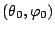. This is done by adding to the total energy one more term,, whereis the Lagrangian multiplier,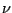is the particle average magnetization direction: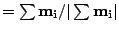,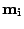is the individual local magnetic spins and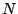is the number of spins inside the particle. This term produces an additional field and, therefore, the total magnetization is biased in the direction. To find the conditional minimum the total system magnetic energy is augmented with the Lagrangian multiplier term and its corresponding effective local field calculated from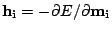. The Landau-Lifshitz-Gilbert equations of motion for each individual spin without the precessional term is solved and concurrently one should add to them also three equations for the Lagrangian multiplier components:. The stationary points found in this approach are also the stationary points of the original Hamiltonian. However, if the system has many metastable states, only part of these points, compatible with the behavior assumed by the biased direction would be found. The method can produce highly non-collinear multidimensional stationary points.The method allows to calculate the effective energy landscape for nanoelements in terms of the biased direction. An example of such a landscape is presented in Fig. 2.12 for a cylindrical magnetic grain, implemented on the basis of micromagnetic model with parameters corresponding to Fe with cubic anisotropy, the exchange parameterand the saturation magnetization value. It is clearly seen that in this case there is a competition between magnetocrystalline and shape anisotropies.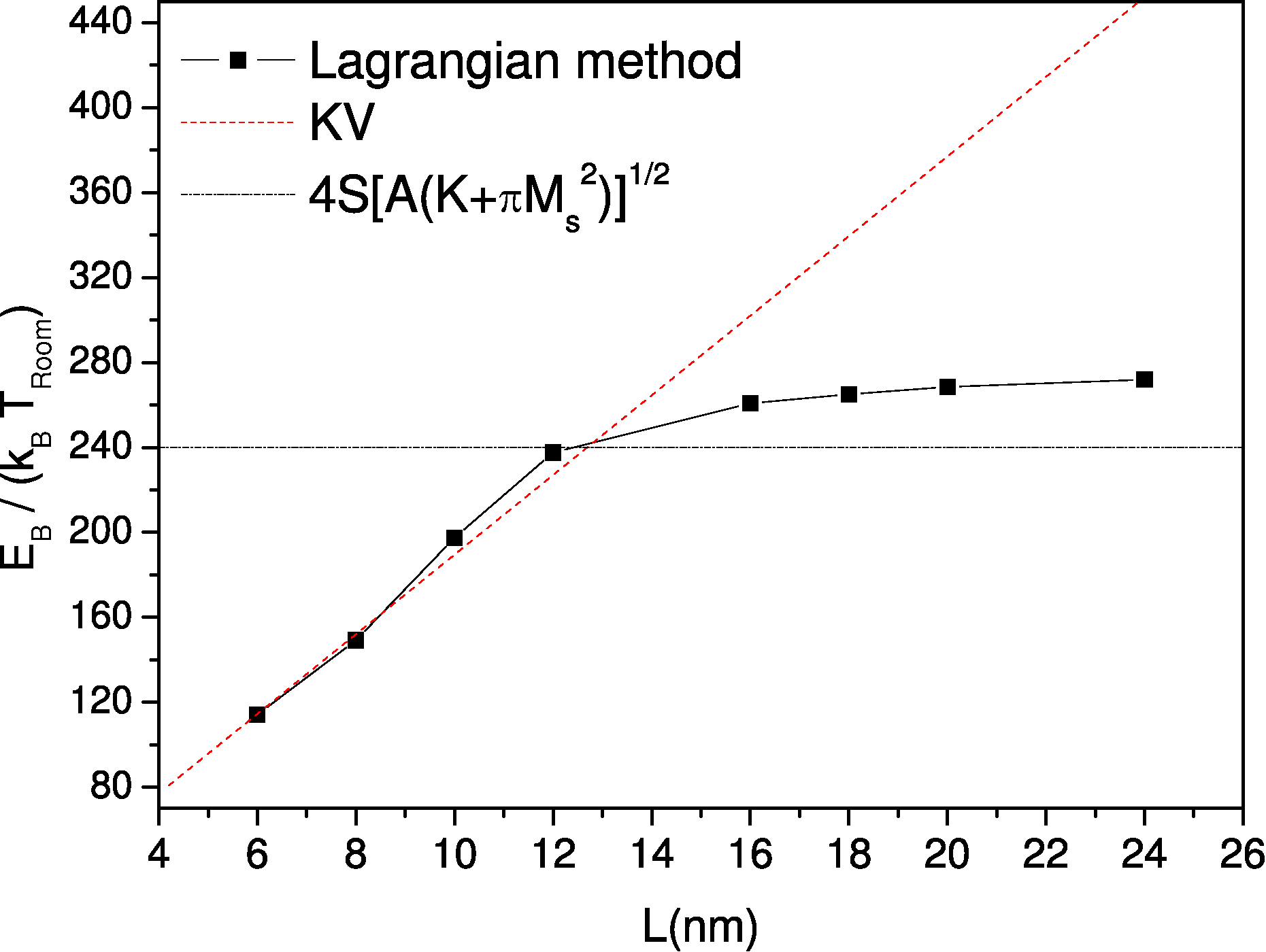The saddle point could be found as the one of the effective energy landscape. Alternatively, we notice that the stationary points coincide with the condition:and, therefore, they can be found minimizing the functional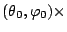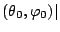, whereis found as a result of the conditional minimization procedure, described above.

To illustrate the performance of the method, we have calculated the energy barriers of FePt rectangular magnetic grains as a function of their elongation. The general idea of these calculations is the same as in Ref. [Forster 03], however, the high-anisotropy grain was implemented on the basis of atomistic calculations with correct lattice structure and the Heisenberg exchange rather than finite element micromagnetic simulations. The parameters used for calculations were that corresponding to FePt: the anisotropy value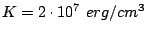and the saturation magnetization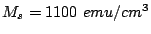, the Heisenberg exchange constant, the lattice parameters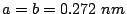and. Fig. 2.13 represents energy barriers of an isolated grain with basis size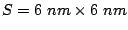as a function of elongationand the corresponding effective energies obtained from the method as shown in Fig. 2.14. The configurations of the saddle points are presented in Figs. 2.15 (a-c). Varying the grain height, we have observed how the configuration of the saddle point changes from that corresponding to coherent rotation (the energy barrier value proportional to system volume) to the one related to the domain wall propagation (the energy barrier value independent on the system volume). The domain wall presence can be observed in Fig. 2.14 as a plateaux in the effective energy because the energy of the domain wall is almost independent of the domain wall center position (as long as its center is far from the grain borders). The critical system size for which the propagation rather than that rotation mode occurs was determined in this case as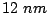. The energy barrier tends to saturate to the domain wall energy. In the absence of the dipolar interaction this energy is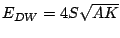. The domain wall energy in this system will be determined mainly by the anisotropy and in order to compare with our results we can correct this value with the contribution of the ideal shape anisotropy of an infinite cylinder but its value has to be calculated numerically. The obtained values are in good agreement with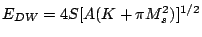as can be seen in Fig. 2.13.We should note here that the determination of the energy barriers in this method is based on the initial guess of the possible mode. The saddle point of the system may be not compatible with the condition assumed by the Lagrangian multiplier term. In practice, the obtained point should be checked to fulfill the condition. In the opposite case the obtained effective energy landscape will not have stationary points. An example of this is the case of two equal grains coupled with phenomenological exchange parameter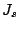. In the small coupling case, the use of the method with one single Lagrangian multiplier produces energy graph with a sharp peak instead of the saddle point. Alternatively, the method fails when the effective energy plot as a function of chosen parametrization coordinates, presents a sudden jump. We can compare these situations to the effective energy plots of Fig. 2.14 where the saddle point is obtained from a continuously differentiable curve. The failure of the method happens often as a consequence of the projection of a multidimensional space to only several degrees of freedom which produces a hysteresis in the energy minimization procedure.

In the case of two weakly coupled grains the best parametrization of the saddle point is the use of two Lagrangian multipliers, adding to the total energy the term: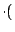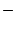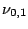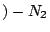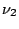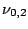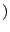, where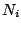is the number of spins in the grain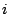and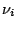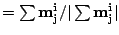is the normalized magnetization vector of the-th grain. This way, we obtain the effective energy contours of two FePt grains of dimension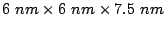as shown in Fig. 2.16 (a-b). For small values of the interfacial exchange parameter, see Fig. 2.16 (a), we can observe the existence of four minima, corresponding to the magnetization of each grain in the two possible opposite directions, which indicates almost independent reversal of the grains. Fig. 2.16 (b) represents the effective energy for values ofclose to full coupling. In this case there are two minima and only one value of energy barrier corresponding to the two equivalent saddle points of the effective energy. Although the contour plots look similar to the case of two interacting magnetic moments, the saddle point configurations correspond to domain-wall structures pinned at the interface similar to Fig. 2.15(c).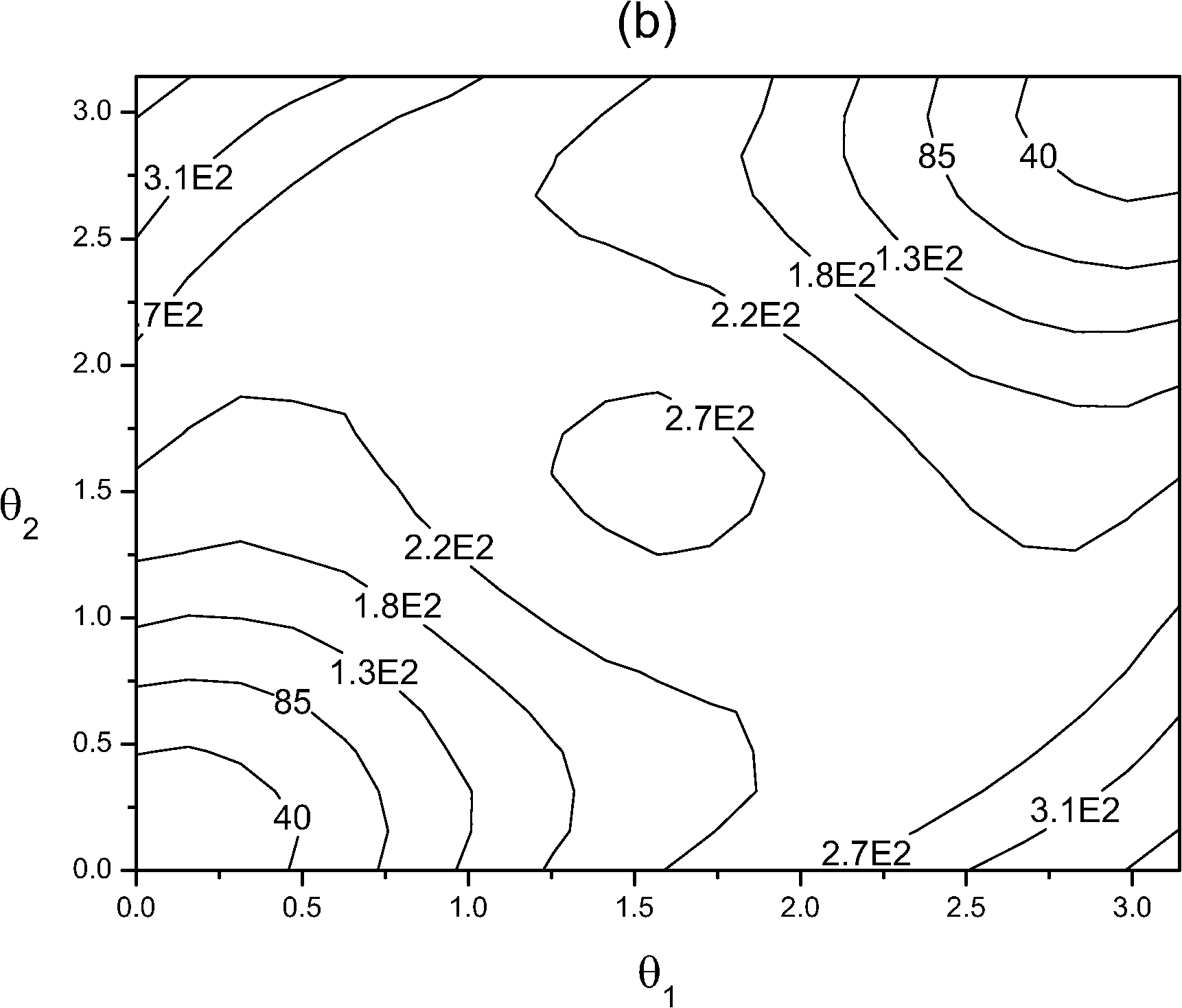In conclusion, the method of the Lagrangian multiplier suggested in
Ref. [Garanin 03] for evaluation of energy barriers in small particles with non-collinear structures has been successfully generalized for more complicated systems such as magnetic grains or dots. The method could be very useful in situations where simple and unique reversal modes are expected and in the case that one can provide a suitable choice of parametrization of complicated multidimensional point in terms of several coordinates. Generally speaking, the same to some extend is true for the elastic band method [Dittrich 02], where one specifies the sense of direction of initial rotation. As for the potential to determine the possible reversal mode, provided that the initial guess is known, the elastic band method seems to be less restrictive. In other complicated situations where many metastable states with possible transitions between them exist, the method suggested in Ref. [Chubykalo-Fesenko 05], which uses the temperature acceleration to produce an initial reversal mode guess, seems to be a good choice.

2008-04-04